# Lesson Notes By Weeks and Term - Primary 3

Weight

WEEK 2&3

Subject: Mathematics

Class: Primary 3

Term: 3rd Term

Topic: Weight

Behavioural Objectives: At the end of the lesson, pupils should be able to:

Mention weight of objects in grams and kilograms

Make meaningful comparison of weight of objects like rocks,minerals.

CONTENT

COMPARING WEIGHT

A bottle of water weigh more than an empty bottle

A sister weighs less than a mug

A mathematics textbook weighs more than an exercise book.

Activity

1. Weigh the objects and record their weights in kilograms or grams.
2. 6 packets of whiteboard markers 2. 3 tins of Milo
3. 1 mathematics textbook 4. 1 bottle of water
4. 10 packets of pencils 6. 1 tin of milk
5. 1 bag of Semolina 8. 1 packet of biscuits
6. 2 big tins of Peak Milk

Standard units of weight

We need to use standard units to measure weight.

The kilogram (kg) is used as a unit when weighing heavy objects like

bags of rice, bags of cement, etc.

The gram (g) is used when weighing lighter objects like pencils, rulers, etc.

Measuring and estimating weight

These are different types of weighing scales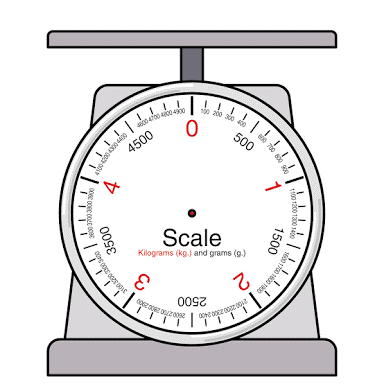Exercise 1

Classify these objects according to their weight in grams and kilograms.

Name of objects� � � � � � Weight in grams (g)���� Weight in kilogram (kg)

1. Bottle of water
2. Pencil
3. Eraser
4. Bag of sugar
5. Jug
6. Ruler
7. Stone
8. Bottle of Fanta

Name of objects ������Weight in grams (g) �����Weight in kilogram (kg)

1. Toothbrush
2. Orange
3. Rock
4. Scissors
5. 5 tins of Milo
6. A bundle of nails
7. 2 notebooks
8. A baby boy

Grams and kilograms

1000 grams (g) = 1 kilogram (kg)

short form short form

We can convert weights in grams to kilograms and weights in kilograms to grams

Examples

2 kg = 1000 g + 1000 g = 2000 g

1/2kg = 1000 g � 2 g = 500 g

2000 g = 2000 g � 1000 g = 2 kg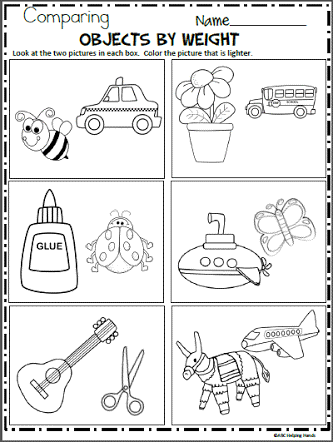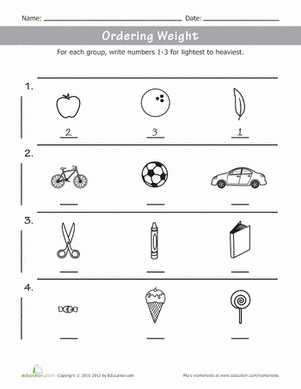Exercise 2

1. How many kilograms are there in these:�

a) 3000 g = b) 2500 g = c) 4000 g =

2. What must I add to 700 g to make 1 kg?

3. How many grams are there in these:

a) 1/2kg = b) 1/4kg = c) 3/4kg =

4. Which is greater?

a) 2000 g or 11/2kg b) 1500 g or 1 kg c) 3500 g or 3 kg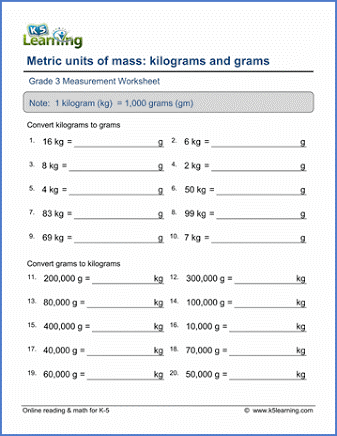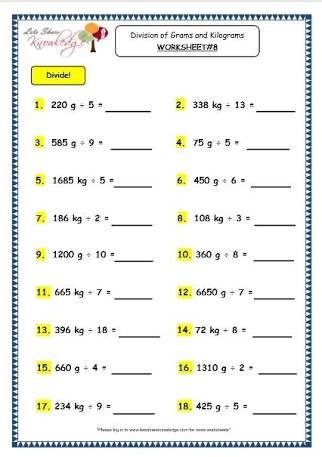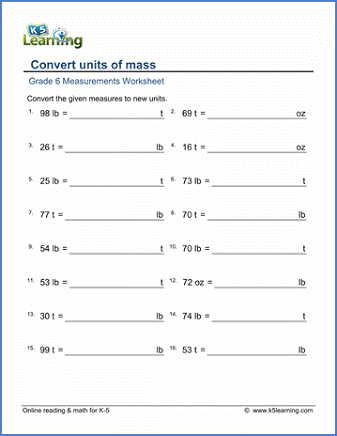1. Copy and complete the following. Use symbols > or <

a) 200 g[] 1/4kg b) 2/5kg[] 300 g c) 100 g[] 1/5kg d) 1 kg[] 700 g

e) 900 g[] 3/4kg f) 400 g[] 1/2kg g) 3/5kg[] 1/2kg h) 3/4kg[] 1/2kg

i) 800 g []3/4kg j) 2/5kg[] 1/4kg

2. Copy and complete the following. Use the number line to help you

a) 1/2kg =[] g b) 3/4kg =[] g c) 1/5kg =[]� g� d) 1/4kg =[] g� e) 2/5kg =[] g� f) 1/10 kg =[] g��� g) 3/5kg =[] g��� h) 4/5kg =[] g

3. Copy and complete the following. The first one is done for you.

a) 11/2kg =[] 1 kg +[] 12kg

= 1 kg + 500 g

= 1 kg 500 g

b) 31/4kg =[] kg +[] g

=[] kg +[] g

c) 1/15kg =[] 1 kg +[] kg

=[] kg[] g

d) 21/2kg =[] kg +[] 12kg=[] kg{} g

e) 23/5kg ={}| kg +{} g

={} kg {}g

f) 3 1/10 kg =() kg +() g

=() kg() g